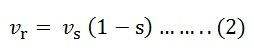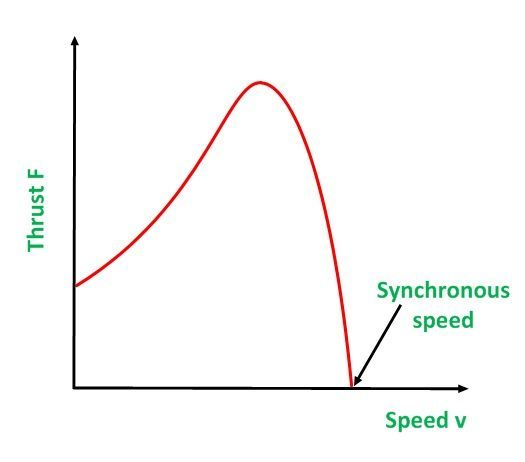# Linear Induction Motor

A Linear Induction Motor (LIM) is an advanced version of rotary induction motor which gives a linear translational motion instead of rotational motion. The stator is cut axially and spread out flat. In this type of motor, the stator and rotor are called primary and secondary, respectively. The secondary of the linear induction motor consists of a flat aluminium conductor with a ferromagnetic core.

The polyphase rotator induction motor is shown below:

If the stator is cut along the line ab and spread out flat the figure formed is shown below. This portion forms the primary of the LIM.If a three-phase supply is connected to the stator of an induction motor, a rotating flux is produced. This flux rotates at a synchronous speed in the air gap. Similarly, if the primary of the linear induction motor is connected to the three-phase supply, a flux is produced which travel across the length of the primary. A current is generated in the conductor which is made of aluminium material.

The current, which is induced in the linear induction motor interacts with the travelling flux produces a linear force. If the secondary of the linear induction motor is fixed and the primary is free to move, the force will move the primary in the direction of the travelling wave.

The double-sided linear induction motor (DLIM) is shown in the figure below:The double-sided linear induction motor has a primary on both sides of the secondary.

## Performance of the Linear Induction Motor

The linear synchronous speed of the travelling wave is given by the equation shown below:Where f is the supply frequency in hertz.

In the rotary induction motor, the speed of the secondary in the linear induction motor is less than the synchronous speed vs and is given as,Where, s is the slip of the linear induction motor and is given as,The linear force is given by the equation shown below:The thrust velocity curve of the linear induction motor is similar to that of the speed torque curve of the rotary induction motor. It is shown in the figure below:If a rotary induction motor is compared with the linear induction motor, the LIM requires a larger air gap and hence, the magnetising current is greater and the power factor and efficiency of the motor are lower. In the rotary induction motor, the stator and the rotor area are the same whereas in the LIM the one of the two is shorter than the other. At the steady speed, the shorter part will be passing continuously over a new part of the other member.

## Applications of the Linear Induction Motor

The various applications of the LIM are as follows:

• The main application of the LIM is in transportation and in electric traction systems. The primary is mounted on the vehicle and the secondary is laid on the track.
• It is used in cranes.
• Pumping of liquid metals.
• Actuators for the movement of doors.
• Used in High voltage circuit breakers and also in accelerators.

This is all about Linear Induction Motor.